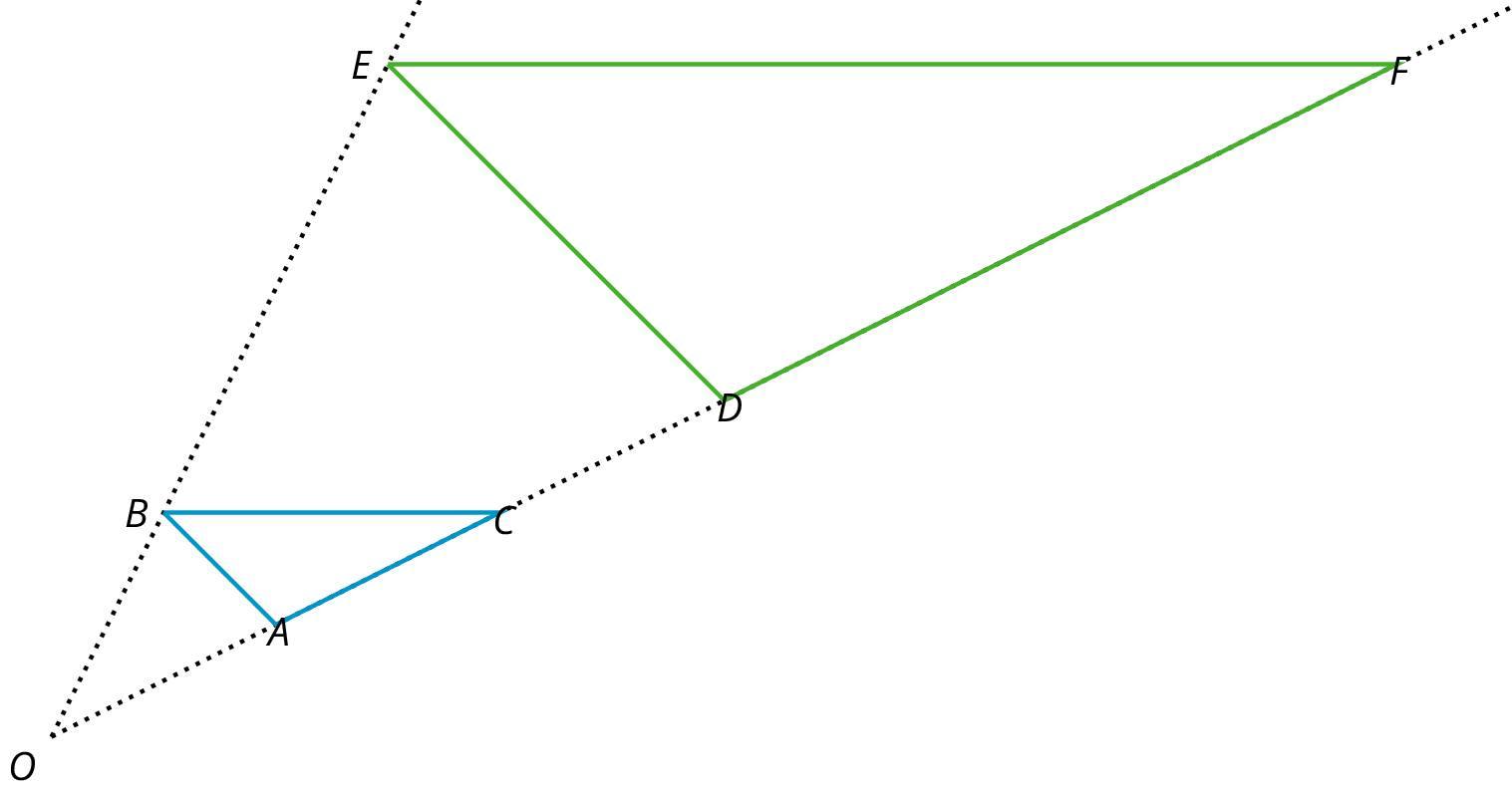# Lesson 9

Dilations

Let’s dilate figures.

### 9.1: Notice and Wonder: Concentric Circles

What do you notice? What do you wonder?

### 9.2: Quadrilateral on a Circular Grid

Here is a polygon $$ABCD$$

1. Dilate each vertex of polygon $$ABCD$$ using $$P$$ as the center of dilation and a scale factor of 2.

2. Draw segments between the dilated points to create a new polygon.
3. What are some things you notice about the new polygon?

4. Choose a few more points on the sides of the original polygon and transform them using the same dilation. What do you notice?

5. Dilate each vertex of polygon $$ABCD$$ using $$P$$ as the center of dilation and a scale factor of $$\frac{1}{2}$$.

Suppose $$P$$ is a point not on line segment $$\overline{WX}$$. Let $$\overline{YZ}$$ be the dilation of line segment $$\overline{WX}$$ using $$P$$ as the center with scale factor 2. Experiment using a circular grid to make predictions about whether each of the following statements must be true, might be true, or must be false.

1. $$\overline{YZ}$$ is twice as long $$\overline{WX}$$.
2. $$\overline{YZ}$$ is five units longer than $$\overline{WX}$$.
3. The point $$P$$ is on $$\overline{YZ}$$.
4. $$\overline{YZ}$$ and $$\overline{WX}$$ intersect.

### 9.3: Getting Perspective

1. Dilate $$P$$ using $$C$$ as the center and a scale factor of 4. Follow the directions to perform the dilations in the applet.
2. Click on the object to dilate, and then click on the center of dilation.
3. When the dialog box opens, enter the scale factor. Fractions can be written with plain text, ex. 1/2.
5. Use the Ray tool and the Distance tool to verify.
2. Dilate $$Q$$ using $$C$$ as the center and a scale factor of $$\frac12$$.

3. Draw a simple polygon.

1. Choose a point outside the polygon to use as the center of dilation. Label it $$C.$$
2. Using your center $$C$$ and the scale factor you were given, draw the image under the dilation of each vertex of the polygon, one at a time. Connect the dilated vertices to create the dilated polygon.
3. Draw a segment that connects each of the original vertices with its image. This will make your diagram look like a cool three-dimensional drawing of a box! If there's time, you can shade the sides of the box to make it look more realistic.
4. Compare your drawing to other people’s drawings. What is the same and what is different? How do the choices you made affect the final drawing? Was your dilated polygon closer to $$C$$ than to the original polygon, or farther away? How is that decided?

Here is line segment $$DE$$ and its image $$D’E’$$ under a dilation.

1. Use a ruler to find and draw the center of dilation. Label it $$F$$.
2. What is the scale factor of the dilation?

### Summary

Dilations also work without a grid. Consider the dilation shown here:

If $$A$$ is the center of dilation, how can we find which point is the dilation of $$B$$ with scale factor 2? Because the scale factor is larger than 1, the point must be farther away from $$A$$ than $$B$$ is, which makes $$C$$ the point we are looking for. If we measure the distance between $$A$$ and $$C$$, we would find that it is exactly twice the distance between $$A$$ and $$B$$.

A dilation with scale factor less than 1 brings points closer. The point $$D$$ is the dilation of $$B$$ with center $$A$$ and scale factor $$\frac{1}{3}$$.

### Glossary Entries

• center of a dilation

The center of a dilation is a fixed point on a plane. It is the starting point from which we measure distances in a dilation.

In this diagram, point $$P$$ is the center of the dilation.

• dilation

A dilation with center $$O$$ and positive scale factor $$r$$ takes a point $$P$$ along the line $$OP$$ to another point whose distance is $$r$$ times further away from $$O$$ than $$P$$ is. If $$r < 1$$ then the new point is really closer to $$O$$, not further away.

The triangle $$DEF$$ is a dilation of the triangle $$ABC$$ with center $$O$$ and with scale factor 3. So $$D$$ is 3 times further away from $$O$$ than $$A$$ is, $$E$$ is 3 times further away from $$O$$ than $$B$$ is, and $$F$$ is 3 times further away from $$O$$ than $$C$$ is.• scale factor

To create a scaled copy, we multiply all the lengths in the original figure by the same number. This number is called the scale factor.

In this example, the scale factor is 1.5, because $$4 \boldcdot (1.5) = 6$$, $$5 \boldcdot (1.5)=7.5$$, and $$6 \boldcdot (1.5)=9$$.